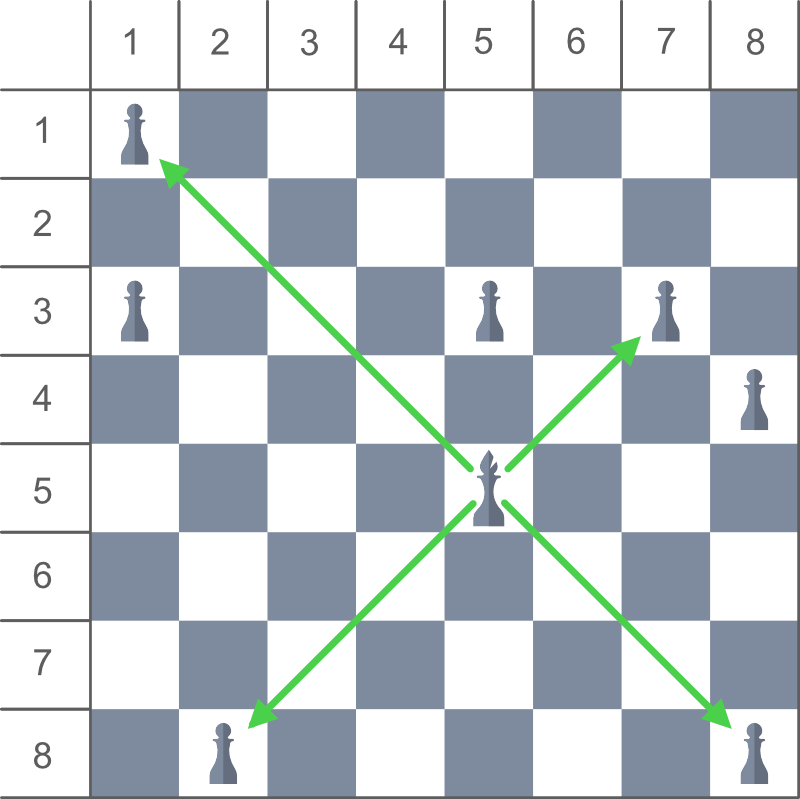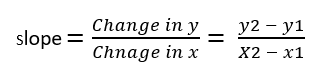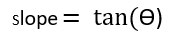# Check whether Bishop can take down Pawn or not

Given the position of a Bishop and a Pawn on a 8 * 8 chessboard, the task is to check whether the Bishop can take down the Pawn in one move or not. The position of Bishop and Pawn is denoted using row and column number of the chessboard.

Examples:

Input: bishopX = 5, bishopY = 5, pawnX = 1, pawnY = 1
Output: Yes

Input: bishopX = 5, bishopY = 5, pawnX = 1, pawnY = 3
Output: No

## Recommended: Please try your approach on {IDE} first, before moving on to the solution.

Approach: In a game of chess, the Bishop can only move diagonally and there is no restriction in the distance for each move. If we consider Bishop’s position as origin, it can be concluded that Bishop can attack at 45 degree, 135 degree, 225 degree and 315 degree angles only. So, the Bishop can attack the Pawn only if they are in such position that the slop obtained from their position is equal to tangent of 45 degree or 135 degree or 225 degree or 315 degree angle.As we know the equation of slope for two points-Also,For all the attacking angles of Bishop (i.e 45°, 135°, 225°, and 315°) value of slope will be either 1 or -1.

tan(45°) = 1
tan(135°) = -1
tan(225°) = 1
tan(315°) = -1

So, for angle 45° and 225°, equation of slope will be

x2 – x1 = y2 – y1

and for angle 135° and 315°, equation of slope will be

-x2 + x1 = y2 – y1

1. Considering the position of Bishop and Pawn as points on 2-D co-ordinate, Check if any of two equation is true
• x2 – x1 = y2 – y1
• -x2 + x1 = y2 – y1
2. Otherwise print No.

Below is the implementation of the above approach:

## C++

 `// C++ implementation of above approach ` `#include ` `using` `namespace` `std; ` ` `  `// Function that return true if ` `// the Bishop can take down the pawn ` `bool` `canTakeDown(``int` `bishopX, ``int` `bishopY, ``int` `pawnX, ``int` `pawnY) ` `{ ` ` `  `    ``// If pawn is at angle ` `    ``// 45 or 225 degree from ` `    ``// bishop's Position ` `    ``if` `(pawnX - bishopX == pawnY - bishopY) ` `        ``return` `true``; ` ` `  `    ``// If pawn is at angle ` `    ``// 135 or 315 degree from ` `    ``// bishop's Position ` `    ``else` `if` `(-pawnX + bishopX == pawnY - bishopY) ` `        ``return` `true``; ` ` `  `    ``else` `        ``return` `false``; ` `} ` ` `  `// Driver code ` `int` `main() ` `{ ` `    ``// Bishop's Position ` `    ``int` `bishopX = 5, bishopY = 5; ` ` `  `    ``// Pawn's Position ` `    ``int` `pawnX = 1, pawnY = 1; ` ` `  `    ``if` `(canTakeDown(bishopX, bishopY, pawnX, pawnY)) ` `        ``cout << ``"Yes"``; ` `    ``else` `        ``cout << ``"No"``; ` `} `

## Java

 `// Java implementation of above approach ` `class` `GFG { ` ` `  `    ``// Function that return true if ` `    ``// the Bishop can take down the pawn ` `    ``static` `boolean` `canTakeDown(``int` `bishopX, ``int` `bishopY, ``int` `pawnX, ``int` `pawnY) ` `    ``{ ` ` `  `        ``// If pawn is at angle ` `        ``// 45 or 225 degree from ` `        ``// bishop's Position ` `        ``if` `(pawnX - bishopX == pawnY - bishopY) ` `            ``return` `true``; ` ` `  `        ``// If pawn is at angle ` `        ``// 135 or 315 degree from ` `        ``// bishop's Position ` `        ``else` `if` `(-pawnX + bishopX == pawnY - bishopY) ` `            ``return` `true``; ` ` `  `        ``else` `            ``return` `false``; ` `    ``} ` ` `  `    ``// Driver code ` `    ``public` `static` `void` `main(String[] args) ` `    ``{ ` `        ``// Bishop's Position ` `        ``int` `bishopX = ``5``, bishopY = ``5``; ` ` `  `        ``// Pawn's Position ` `        ``int` `pawnX = ``1``, pawnY = ``1``; ` ` `  `        ``if` `(canTakeDown(bishopX, bishopY, pawnX, pawnY)) ` `            ``System.out.println(``"Yes"``); ` `        ``else` `            ``System.out.println(``"No"``); ` `    ``} ` `} `

## Python3

 `# Python3 implementation of above approach ` ` `  `# Function that return true if  ` `# the Bishop can take down the pawn ` `def` `canTakeDown(bishopX, bishopY, pawnX, pawnY) : ` ` `  ` `  `    ``# If pawn is at angle  ` `    ``# 45 or 225 degree from ` `    ``# bishop's Position ` `    ``if` `(pawnX ``-` `bishopX ``=``=` `pawnY ``-` `bishopY) : ` `        ``return` `True` `     `  `    ``# If pawn is at angle  ` `    ``# 135 or 315 degree from ` `    ``# bishop's Position ` `    ``elif` `(``-``pawnX ``+` `bishopX ``=``=` `pawnY ``-` `bishopY): ` `        ``return` `True` `     `  `    ``else``: ` `        ``return` `False` ` `  ` `  `# Driver code ` ` `  `# Bishop's Position ` `bishopX ``=` `5` `bishopY ``=` `5` ` `  `# Pawn's Position ` `pawnX ``=` `1` `pawnY ``=` `1` ` `  `if` `(canTakeDown(bishopX, bishopY, pawnX, pawnY)) : ` `    ``print``(``"Yes"``) ` `else` `: ` `    ``print``(``"No"``) `

## C#

 `// C# implementation of above approach ` `using` `System; ` `class` `GFG { ` ` `  `    ``// Function that return true if ` `    ``// the Bishop can take down the pawn ` `    ``static` `bool` `canTakeDown(``int` `bishopX, ``int` `bishopY, ``int` `pawnX, ``int` `pawnY) ` `    ``{ ` ` `  `        ``// If pawn is at angle ` `        ``// 45 or 225 degree from ` `        ``// bishop's Position ` `        ``if` `(pawnX - bishopX == pawnY - bishopY) ` `            ``return` `true``; ` ` `  `        ``// If pawn is at angle ` `        ``// 135 or 315 degree from ` `        ``// bishop's Position ` `        ``else` `if` `(-pawnX + bishopX == pawnY - bishopY) ` `            ``return` `true``; ` ` `  `        ``else` `            ``return` `false``; ` `    ``} ` ` `  `    ``// Driver code ` `    ``public` `static` `void` `Main() ` `    ``{ ` `        ``// Bishop's Position ` `        ``int` `bishopX = 5, bishopY = 5; ` ` `  `        ``// Pawn's Position ` `        ``int` `pawnX = 1, pawnY = 1; ` ` `  `        ``if` `(canTakeDown(bishopX, bishopY, pawnX, pawnY)) ` `            ``Console.WriteLine(``"Yes"``); ` `        ``else` `            ``Console.WriteLine(``"No"``); ` `    ``} ` `} `

## PHP

 ` `

Output:

```Yes
```

My Personal Notes arrow_drop_upCheck out this Author's contributed articles.

If you like GeeksforGeeks and would like to contribute, you can also write an article using contribute.geeksforgeeks.org or mail your article to contribute@geeksforgeeks.org. See your article appearing on the GeeksforGeeks main page and help other Geeks.

Please Improve this article if you find anything incorrect by clicking on the "Improve Article" button below.

Improved By : jit_t